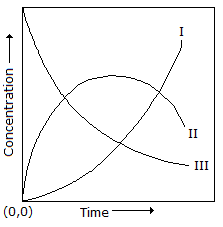# Chemical Engineering - Mass Transfer

1.

Which of the following is a suitable absorbent for removal of H2S from natural gas ?

 A. Vetrocoke solution B. Monoethanol amine (MEA) C. Dilute D. Hot water

Answer: Option B

Explanation:

No answer description available for this question. Let us discuss.

2.

Acetone is to be removed from air in an isothermal dilute absorber using pure water as solvent. The incoming air contains 5 mole% of acetone (yin = 0.05). The design equation to be used for obtaining the number of trays (N) of the absorber is, N+2 = 6 log (yin/yout).For 98% recovery of acetone, the number of trays required is/are

 A. 1 B. 8 C. 9 D. 10

Answer: Option C

Explanation:

No answer description available for this question. Let us discuss.

3.

Schimdt number and Lewis number for pure air at atmospheric conditions are respectively

 A. < 1 and ≈ 1 B. 1 and 0 C. >1 and ≈ 1 D. 0 and ≈ 1

Answer: Option A

Explanation:

No answer description available for this question. Let us discuss.

4.

Below is given an equilibrium moisture curve of a substance. State which regions represent free moisture and unbound moisture respectively.A. II, III B. II, IV C. IV, III D. IV, II

Answer: Option A

Explanation:

No answer description available for this question. Let us discuss.

5.

Molecular diffusion is caused by the

 A. transfer of molecules from low concentration to high concentration region. B. thermal energy of the molecules. C. activation energy of the molecules. D. potential energy of the molecules.

Answer: Option B

Explanation:

No answer description available for this question. Let us discuss.

#### Current Affairs 2021

Interview Questions and Answers